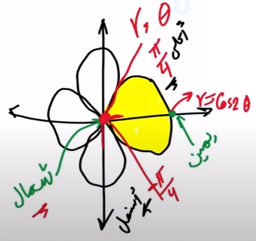Need Help?

Subscribe to Calculus C

###### \${selected_topic_name}
• Notes
• Comments & Questions

Use a double integral to find the area enclosed by one loop of the four-
leaved rose $r=\cos 2 \theta .$

Area $=\int_{y} \int_{x} d x d y=\int_{r_{1}}^{r_{2}} \int_{\theta_{1}}^{\theta_{2}} r d r d \theta$$\int_{-{\pi/4}}^{\pi/4} \int_{0}^{\cos 2 \theta} r d r d \theta$

$=\int_{-\frac{\pi}{4}}^\frac {\pi}{4}\left[\int_{0}^{\cos 2 \theta} r d r\right] d \theta$

$=\int_{-\frac{\pi}{4}}^{\frac {\pi}{4}} \int_{0}^{\cos 2 \theta} \frac{r^{2}}{2} d\theta$

$=\int_{-\frac{\pi}{4}}^{\frac{\pi}{4}} \frac{\cos (2 \theta)^{2}}{2}=\frac{\pi}{8}$

No comments yet